# Business and Finance Math #4: Continuous Compounding on the TI BA II Plus & HP 12c

Filed under Difficulty: Easy, Finance, HP 12c, TI BA II Plus.

Sometimes when reviewing time value of money (TVM) problems, you may encounter a situation that involves continuous compounding. As we have seen in our previous posts on interest rates and calculating effective rates, the more times compounding occurs, the higher the effective rate, and the more you will earn on your investment or bank account (or pay on a loan). This naturally leads to the question: what is the maximum benefit you can receive from compounding? Enter continuous compounding, where compounding occurs constantly. As soon as interest is earned on an investment, it is immediately compounded. Keep reading to learn how to solve problems with continuous compounding on your TI BA II Plus or HP 12c financial calculator.

### Mathematical Background

The following table shows how the effective annual interest rate increases with the frequency of compoundings:

 Period # Compoundings / Year EAR Year 1 8.00000% Semiannual 2 8.16000% Quarter 4 8.24322% Month 12 8.29995% Week 52 8.32205% Day 365 8.32776% Hour 8,760 8.32867% Minute 525,600 8.32871%

The more times a given rate (in this case, 8%) is compounded, the effective annual interest rate increases, but only to a certain point. As you can see, there was very little change in the EAR when we increased the compounding from an hourly basis to compounding by the minute.

In mathematical terms, we can say that the EAR approaches a limit, or maximum value, as we increase the number of times compounding occurs. In the table above, as we increase the number of times 8% is compounded per year, we grow closer to or approach an interest rate of approximately 8.33%.

Our goal is to calculate the interest rate with continuous compounding, where interest is compounded as soon as it is earned. To do this, we keep increasing the number of compoundings towards positive infinity (a higher and higher number of compoundings). Eventually, there will be no or very little change in the interest rate as we increase the number of times compounding occurs.

The general formula we are going to use for determining the effective annual rate is as follows: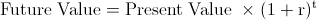This formula calculates the size of an investments after a certain number of years t for a given interest rate represented by r. We can modify this equation to account for multiple compoundings in a given year: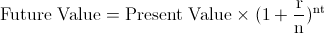Here, we divide the interest rate r by n, which represents the number of compoundings per year. We can then use this equation to find how large \$100 would grow over 1 year at an interest rate of 8% compounded quarterly: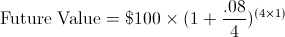Here we take the interest rate r of 8% and divide it by 4, which represents n, the 4 times per year that interest is compounded. After adding 1 to this expression, we raise it to 4 × 1, representing 4 for n and 1 for t, the number of years. After one year with quarterly compounding, \$100 invested at 8% will grow to be\$108.24. If you refer to the table earlier in this post, you can see that an interest rate of 8% compounded quarterly is equal to about 8.24%.

Here is what happens to the effective interest rate as we keep increasing the number of times compounding occurs each year:

 Period Periods / Year Rate/Period (r/n) EAR Year 1 .08/1 8.00000% Semiannual 2 .08/2 8.16000% Quarter 4 .08/4 8.24322% Month 12 .08/12 8.29995% Week 52 .08/52 8.32205% Day 365 .08/365 8.32776%

Mathematically, we can express larger and larger values for n (the number of compoundings) as a limit: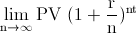As n grows larger and larger, this limit turns out to be: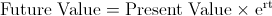e is a mathematical constant (also called Euler’s Number) which also appears in many other areas of mathematics and science, and is approximately equal to 2.71828.

This formula for finding the future value of an initial investment that is continuously compounded can be manipulated to yield the following formula that we can use for calculating the effective interest rate: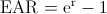Where r is your stated interest rate. Now let’s see how we can solve continuous compounding problems on our financial calculator! The question we are going to answer is:

What is the effective rate of 8% with continuous compounding?

### Continuous Compounding on the TI BA II Plus

The steps to determine the effective rate of 8% compounded continuously are as follows:

1. Press followed by 2nd to select ex
2. Next press and you will have the effective interest rate on your screen

The correct answer is approximately 8.3287%

### Continuous Compounding on the HP 12c

1. Set the 12c to RPN mode by pressing f if it is not already set
2. Enter the interest rate by entering
3. Next press g to select ex, followed by

You should see the effective rate of 8.3287% on the calculator’s screen.

Experiment with different interest rates and see the rate you would really earn with continuous compounding!

### 3 Responses to Business and Finance Math #4: Continuous Compounding on the TI BA II Plus & HP 12c

1.Doug Wood

Hi-
Great article and helpful for my limited math skills. I have a question.
I’m looking at automatic reinvested dividends (DRIP’s) BUT the dividends are not just 4% but growing by 10%/yr…..compound interest with increasing interest rates!
Add to that the increase in annual value of a stock at 6% gains/yr.
I have looked everywhere for an equation and steps on the HP to calculate this and even seen article that show the returns on this strategy over 25 yrs. but no formula to calculate it.
Thanks tons,
Doug

2.Doug Wood

Hi-I’ll try again and hope the Yahoo acct. is right.

Great article and helpful for my limited math skills. I have a question.
I’m looking at automatic reinvested dividends (DRIP’s) BUT the dividends are not just 4% but growing by 10%/yr…..compound interest with increasing interest rates!
Add to that the increase in annual value of a stock at 6% gains/yr.
I have looked everywhere for an equation and steps on the HP to calculate this and even seen article that show the returns on this strategy over 25 yrs. but no formula to calculate it.
Thanks tons,
Doug

3.Quentin

Thank you for this post. Very helpful and informative.